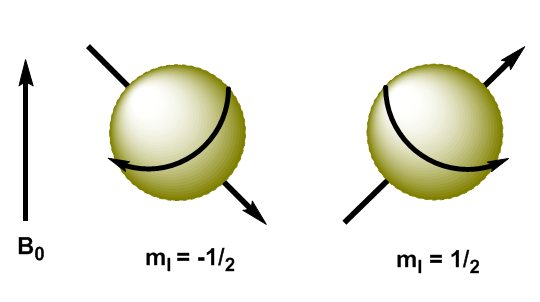Atomic nuclei are charged particles and their angular momentum produces magnetic momentum, represented by $\mu$ \begin{equation}\label{ec3} \mu=\gamma L = \gamma \sqrt{I(I+1)} \hbar \end{equation} where $\gamma$ is the gyromanetic constant, characteristic of each nucleus. When we apply a magnetic field, $B_0$, to nuclei with I=1/2, the magnetic moments are oriented in such a way that the nuclei with $m_I=1/2$ have their magnetic moment aligned with the field and the nuclei with $m_I=-1/2$ have their magnetic moment opposite to the applied field.